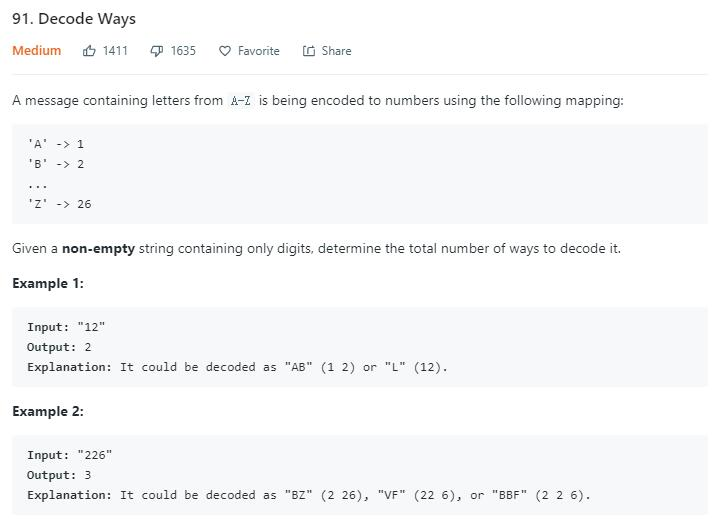# 題目描述（中等难度）# 解法一 递归

2|32232323232 和 23|2232323232

public int numDecodings(String s) {
return getAns(s, 0);
}

private int getAns(String s, int start) {
//划分到了最后返回 1
if (start == s.length()) {
return 1;
}
//开头是 0,0 不对应任何字母，直接返回 0
if (s.charAt(start) == '0') {
return 0;
}
//得到第一种的划分的解码方式
int ans1 = getAns(s, start + 1);
int ans2 = 0;
//判断前两个数字是不是小于等于 26 的
if (start < s.length() - 1) {
int ten = (s.charAt(start) - '0') * 10;
int one = s.charAt(start + 1) - '0';
if (ten + one <= 26) {
ans2 = getAns(s, start + 2);
}
}
return ans1 + ans2;
}


# 解法二 递归 memoization

public int numDecodings(String s) {
HashMap<Integer, Integer> memoization = new HashMap<>();
return getAns(s, 0, memoization);
}

private int getAns(String s, int start, HashMap<Integer, Integer> memoization) {
if (start == s.length()) {
return 1;
}
if (s.charAt(start) == '0') {
return 0;
}
//判断之前是否计算过
int m = memoization.getOrDefault(start, -1);
if (m != -1) {
return m;
}
int ans1 = getAns(s, start + 1, memoization);
int ans2 = 0;
if (start < s.length() - 1) {
int ten = (s.charAt(start) - '0') * 10;
int one = s.charAt(start + 1) - '0';
if (ten + one <= 26) {
ans2 = getAns(s, start + 2, memoization);
}
}
//将结果保存
memoization.put(start, ans1 + ans2);
return ans1 + ans2;
}


# 解法三 动态规划

dp [ i ] = dp[ i + 1 ] + dp [ i + 2 ]

public int numDecodings(String s) {
int len = s.length();
int[] dp = new int[len + 1];
dp[len] = 1; //将递归法的结束条件初始化为 1
//最后一个数字不等于 0 就初始化为 1
if (s.charAt(len - 1) != '0') {
dp[len - 1] = 1;
}
for (int i = len - 2; i >= 0; i--) {
//当前数字时 0 ，直接跳过，0 不代表任何字母
if (s.charAt(i) == '0') {
continue;
}
int ans1 = dp[i + 1];
//判断两个字母组成的数字是否小于等于 26
int ans2 = 0;
int ten = (s.charAt(i) - '0') * 10;
int one = s.charAt(i + 1) - '0';
if (ten + one <= 26) {
ans2 = dp[i + 2];
}
dp[i] = ans1 + ans2;

}
return dp;
}


public int numDecodings4(String s) {
int len = s.length();
int[] dp = new int;
dp[len % 3] = 1;
if (s.charAt(len - 1) != '0') {
dp[(len - 1) % 3] = 1;
}
for (int i = len - 2; i >= 0; i--) {
if (s.charAt(i) == '0') {
dp[i % 3] = 0; //这里很重要，因为空间复用了，不要忘记归零
continue;
}
int ans1 = dp[(i + 1) % 3];
int ans2 = 0;
int ten = (s.charAt(i) - '0') * 10;
int one = s.charAt(i + 1) - '0';
if (ten + one <= 26) {
ans2 = dp[(i + 2) % 3];
}
dp[i % 3] = ans1 + ans2;

}
return dp;
}


public int numDecodings5(String s) {
int len = s.length();
int end = 1;
int cur = 0;
if (s.charAt(len - 1) != '0') {
cur = 1;
}
for (int i = len - 2; i >= 0; i--) {
if (s.charAt(i) == '0') {
end = cur;//end 前移
cur = 0;
continue;
}
int ans1 = cur;
int ans2 = 0;
int ten = (s.charAt(i) - '0') * 10;
int one = s.charAt(i + 1) - '0';
if (ten + one <= 26) {
ans2 = end;
}
end = cur; //end 前移
cur = ans1 + ans2;

}
return cur;
}